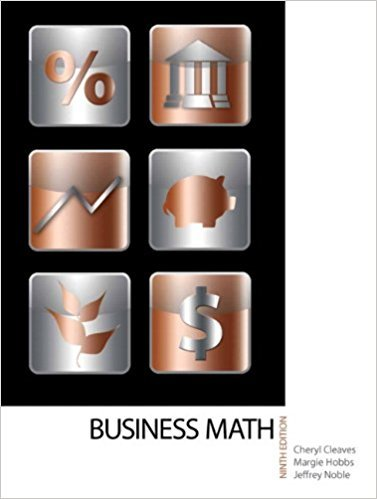×
×

# Solutions for Chapter 10-1: GROSS PAY## Full solutions for Business Math, | 9th Edition

ISBN: 9780135108178Solutions for Chapter 10-1: GROSS PAY

Solutions for Chapter 10-1
4 5 0 400 Reviews
12
5
##### ISBN: 9780135108178

This expansive textbook survival guide covers the following chapters and their solutions. This textbook survival guide was created for the textbook: Business Math, , edition: 9. Since 16 problems in chapter 10-1: GROSS PAY have been answered, more than 17375 students have viewed full step-by-step solutions from this chapter. Business Math, was written by and is associated to the ISBN: 9780135108178. Chapter 10-1: GROSS PAY includes 16 full step-by-step solutions.

Key Math Terms and definitions covered in this textbook
• Adjacency matrix of a graph.

Square matrix with aij = 1 when there is an edge from node i to node j; otherwise aij = O. A = AT when edges go both ways (undirected). Adjacency matrix of a graph. Square matrix with aij = 1 when there is an edge from node i to node j; otherwise aij = O. A = AT when edges go both ways (undirected).

• Back substitution.

Upper triangular systems are solved in reverse order Xn to Xl.

• Circulant matrix C.

Constant diagonals wrap around as in cyclic shift S. Every circulant is Col + CIS + ... + Cn_lSn - l . Cx = convolution c * x. Eigenvectors in F.

A sequence of steps (end of Chapter 9) to solve positive definite Ax = b by minimizing !x T Ax - x Tb over growing Krylov subspaces.

• Determinant IAI = det(A).

Defined by det I = 1, sign reversal for row exchange, and linearity in each row. Then IAI = 0 when A is singular. Also IABI = IAIIBI and

• Echelon matrix U.

The first nonzero entry (the pivot) in each row comes in a later column than the pivot in the previous row. All zero rows come last.

• Full row rank r = m.

Independent rows, at least one solution to Ax = b, column space is all of Rm. Full rank means full column rank or full row rank.

• Jordan form 1 = M- 1 AM.

If A has s independent eigenvectors, its "generalized" eigenvector matrix M gives 1 = diag(lt, ... , 1s). The block his Akh +Nk where Nk has 1 's on diagonall. Each block has one eigenvalue Ak and one eigenvector.

• Kronecker product (tensor product) A ® B.

Blocks aij B, eigenvalues Ap(A)Aq(B).

• Krylov subspace Kj(A, b).

The subspace spanned by b, Ab, ... , Aj-Ib. Numerical methods approximate A -I b by x j with residual b - Ax j in this subspace. A good basis for K j requires only multiplication by A at each step.

• Least squares solution X.

The vector x that minimizes the error lie 112 solves AT Ax = ATb. Then e = b - Ax is orthogonal to all columns of A.

• Left inverse A+.

If A has full column rank n, then A+ = (AT A)-I AT has A+ A = In.

• Nilpotent matrix N.

Some power of N is the zero matrix, N k = o. The only eigenvalue is A = 0 (repeated n times). Examples: triangular matrices with zero diagonal.

• Norm

IIA II. The ".e 2 norm" of A is the maximum ratio II Ax II/l1x II = O"max· Then II Ax II < IIAllllxll and IIABII < IIAIIIIBII and IIA + BII < IIAII + IIBII. Frobenius norm IIAII} = L La~. The.e 1 and.e oo norms are largest column and row sums of laij I.

• Outer product uv T

= column times row = rank one matrix.

• Polar decomposition A = Q H.

Orthogonal Q times positive (semi)definite H.

• Projection matrix P onto subspace S.

Projection p = P b is the closest point to b in S, error e = b - Pb is perpendicularto S. p 2 = P = pT, eigenvalues are 1 or 0, eigenvectors are in S or S...L. If columns of A = basis for S then P = A (AT A) -1 AT.

• Semidefinite matrix A.

(Positive) semidefinite: all x T Ax > 0, all A > 0; A = any RT R.

• Skew-symmetric matrix K.

The transpose is -K, since Kij = -Kji. Eigenvalues are pure imaginary, eigenvectors are orthogonal, eKt is an orthogonal matrix.

• Symmetric factorizations A = LDLT and A = QAQT.

Signs in A = signs in D.

×